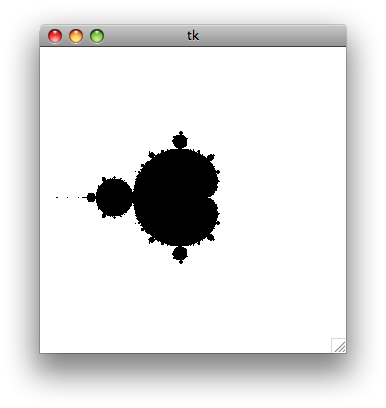Simple Mandelbrot generator using Python

16 mai 2008

I was looking for a programming language for beginners, that I could teach to my little sister — something different from Basic, which is a bit outdated. I finally looked at Python, and I wrote a small Fractal generator for testing purpose. As I didn’t found a simple example on the Internet, I give my version here.

It is only a simple example, which generate the Mandelbrot fractal. At first, no zoom, no colors — I implemented that later, but here is the first and simplest version. And it works on Mac and Windows, of course.And here is the code :

[python]from Tkinter import *# Global variablescwidth = 300         # Width of the canvascheight = 300        # Height of the canvasloops = 200          # Maximum number of iterations# Mandelbrot function# cv : canvas# x1, y1 : coordinates of the uper left point of the window# x2, y2 : coordinates of the bottom right point of the windowdef mandel(cv, x1, y1, x2, y2):    # Compute the x and y increment    dx = float(abs(x2 - x1)) / cwidth    dy = float(abs(y2 - y1)) / cheight        # Let's start the maths    y = y1    for j in range(0, cheight):        x = x1        for i in range(0, cwidth):                        x = x + dx            c = complex(x, y)            a = 0            # Core loop : x = x^2 + c, loop n times,            # and see if the number escape from a circle centered on 0            for k in range(0, loops):                a = a*a + c                if abs(a) > 4:                    break                        # Draw a plot if we didn't escape the loop            if k == (loops - 1):                cv.create_line(i, j, i, j + 1)                    y = y - dy        cv.update()# Create window and canvasroot = Tk()c = Canvas(root,width = cwidth, height = cheight)c.pack()# Draw the Mandelbrot fractalmandel(c, -2, 2, 2, -2)# Run event looproot.mainloop()

Next week we’ll see how to add some colors and zoom support without hassle.

Discussion, liens, et tweetsJ’écris des sites web, des logiciels, des applications mobiles. Vous me trouverez essentiellement sur ce blog, mais aussi sur Mastodon, parmi les Codeurs en Liberté, ou en haut d’une colline du nord-est de Paris.